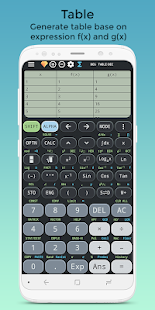Features
☆ Basic Calculator: calculate from basic mathematics functions with percentage to advanced functions.
☆ Scientific Calculator: multi-functional scientific calculator with a lot of functions, all in one app.
☆ Equation Solver: Solve quadratic, cubic, quartic and other complex math equations.
☆ Camera Math: Enter math expression by using camera get answer.
☆ Calculation History: save your calculation history.
☆ Base-N calculation for programmer
☆ Integral calculator provide powerful calculator to calculate integrate, derivative

◉ Math natural display
See math expressions, symbols and fractions exactly the way they appear in textbooks.

◉ Scientific calculator
This Scientific Calculator contains all the scientific calculations such as equation solver, derivative, anti-trigonometric calculation, trigonometric calculation, integral, logarithmic calculation, square root calculation, power calculation, factorial calculation, cube root calculation, π calculation and so on.

◉ Photo calculator – Scan Math Problems and Solve by Camera
Photo calculator is the most creative feature of this calculator. This feature solves problem by taking photo, inputs quickly to solve math with your camera. Unlike the other calculator, this calculator help you solve math problem quickly by taking a picture and then you can get the solutions at once.

◉ Real simulator: calculator layout same as calculator 570 es plus and 991 fx es plus as well as 580 ex plus. It also supports most of features of ti 34, ti 36X Pro, ti 30Xa, texas & instruments calculator ti30, ti 84 plus, ti 83 Plus, ti 89. Wabbit calculator & wabbitemu calculator, grafncalc83, graphncalc83

◉ Graphing – simulates for graphing calculator ti 83 and ti 84
This calculator can draw simultaneously graph, this is the best way to visual functions

◉ Unit conversion
A large collection of unit conversion, include real time currency converter and a lot of units such as time, length, area, …

◉ Calculation formulas
Contains Math formulas and Physics formulas, very efficient for students in high school or university.

◉ Programming – the programmable calculator
Mathematica language is also supported by this programming calculator

Mod Info:
Disabled / Removed unwanted Permissions + Receivers and Services;
Analytics / Crashlytics disabled.

Screenshots

•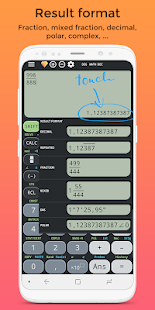•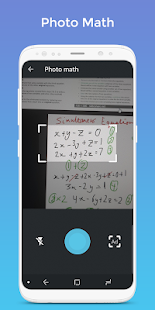•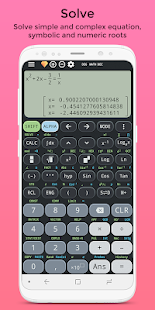•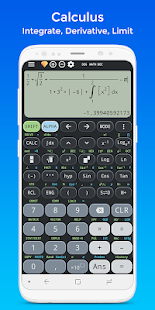•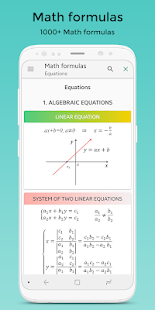•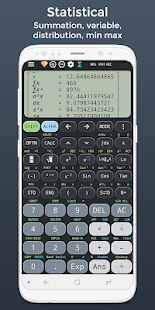•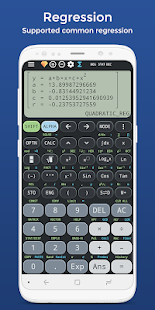•